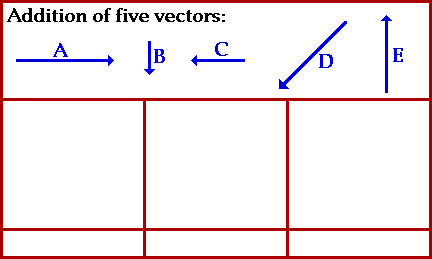## Vector Addition: The Order Does NOT Matter

The summative result of two or more vectors can be determined by a process of vector addition. The most common method of adding vectors is the graphical method of head-to-tail addition. This method involves the selection of a scale (e.g., 1 cm = 5 km), and the subsequent drawing of each vector to scale in the specific direction. The tail of each consecutive vector begins at the head of the most recent vector. The resultant vector (the summative result of the addition of the given vectors) is then drawn from the tail of the first vector to the head of the last vector. The magnitude and direction of the resultant is then determined using a protractor, ruler, and the indicated scale.

The question often arises as to the importance of the order in which the vectors are added. For instance, if five vectors are added - let's call them vectors A, B, C, D and E - then will a different resultant be obtained if a different order of addition is used. Will A + B + C + D + E yield the same result as C + B + A + D + E or D + E + A + B + C? The animation below provides the answer. Observe the animation a couple of times and see what the answer is.As shown in the animation above, the order in which two or more vectors are added does not effect the outcome. Adding A + B + C + D + E yields the same result as adding C + B + A + D + E or D + E + A + B + C! The resultant, shown as the green vector, has the same magnitude and direction regardless of the order in which the five individual vectors are added.

For more information on physical descriptions of motion, visit The Physics Classroom Tutorial. Detailed information is available there on the following topics:

Vectors and Direction